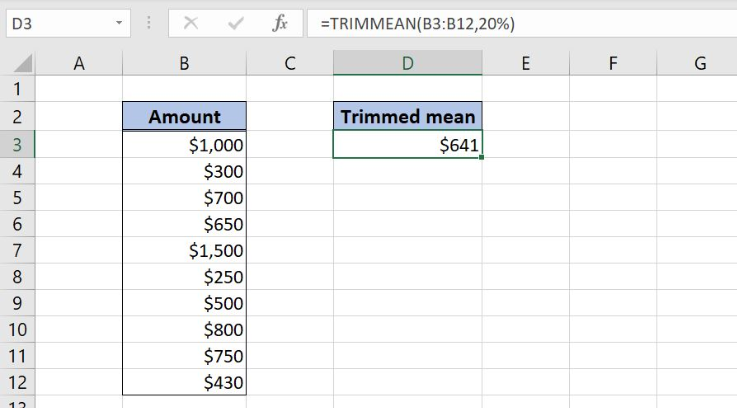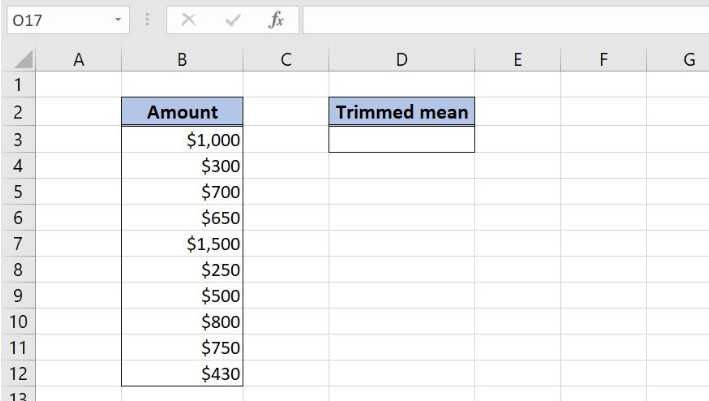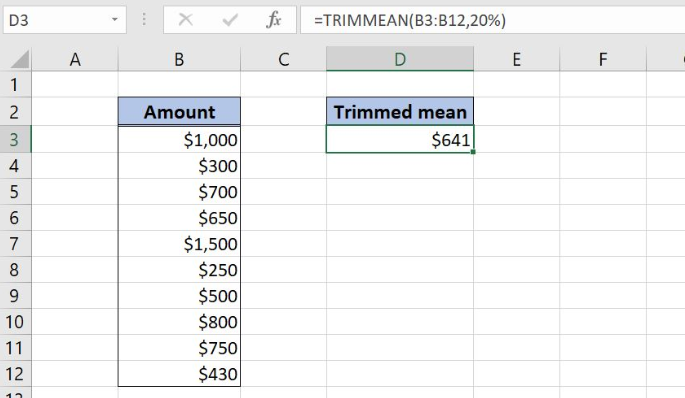Get instant live expert help with Excel or Google Sheets“My Excelchat expert helped me in less than 20 minutes, saving me what would have been 5 hours of work!”

#### Post your problem and you’ll get Expert help in seconds.

Your message must be at least 40 characters
Our professional Expert are available now. Your privacy is guaranteed.

# Excel TRIMMEAN Function

Excel allows us to calculate a trimmed mean for a list of values, using the TRIMMEAN function. A trimmed mean is the average of values, excluding a certain number of highest and lowest values. This step by step tutorial will assist all levels of Excel users in finding trimmed mean for a list of values.Figure 1. The final result of the TRIMMEAN function

## Syntax of the TRIMMEAN formula

`=TRIMMEAN(array, percent)`

The parameters of the TRIMMEAN function are:

• array – a list of values for which we want to calculate trimmed mean
• percent – a percentage of values that we want to exclude from the calculation. Half of them is excluded from the top and another half from the bottom.

## Setting up Our Data for the TRIMMEAN Function

In column B (“Amount”), we have the list of values for which we want to find the trimmed mean. In the cell D3, we want to get the result of the function.Figure 2. Data that we will use in the TRIMMEAN example

## Calculate the Trimmed Mean for the List of Values

In the cell D3, we want to get the trimmed mean for the range B3:B12. We exclude 20% of the values.

The formula looks like:

`=TRIMMEAN(B3:B12, 20%)`

The array is B3:B12, while the percent is 20%.

To apply the TRIMMEAN function, we need to follow these steps:

• Select cell D3 and click on it
• Insert the formula: `=TRIMMEAN(B3:B12, 20%)`
• Press enter.Figure 3. Using the TRIMMEAN function to get a trimmed mean for the list of values

As we can see, there are 10 values in column B and we want to exclude 20% of them, which is 2. This means that the function will exclude one value from the top (\$1,500) and one value from the bottom (\$250). The trimmed mean for the list in the cell D3 in \$641.

Most of the time, the problem you will need to solve will be more complex than a simple application of a formula or function. If you want to save hours of research and frustration, try our live Excelchat service! Our Excel Experts are available 24/7 to answer any Excel question you may have. We guarantee a connection within 30 seconds and a customized solution within 20 minutes.